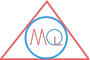# Profit and Loss Formulas

## Profit, loss, selling price, cost price, marked price and discount formulas

### Abrreviations used in the following formulas:

SP $$\implies$$ selling price
CP $$\implies$$ cost price
MP $$\implies$$ marked price
d $$\implies$$ discount
$$d_1$$ $$\implies$$ first discount %age
$$d_2$$ $$\implies$$ second discount %age
$$d_3$$ $$\implies$$ third discount %age

$$Profit = SP \; – \;CP$$ $$Loss = CP \;-\; SP$$ $$Profit\; \% = \frac{Profit}{CP} \times 100$$ $$Loss \;\% = \frac{Loss}{CP} \times 100$$ $$SP = (\frac{100 \;+\; Profit \;\%}{100}) \times CP$$ $$CP = (\frac{100}{100 \;+\; Profit \;\%}) \times SP$$ $$SP = (\frac{100 \;- \;Loss \;\%}{100}) \times CP$$ $$CP = (\frac{100}{100 \;- \;Loss \;\%}) \times SP$$ $$Discount = MP \;-\; SP$$ $$SP = MP \;-\; Discount$$ $$MP = SP \;+\; Discount$$ $$SP = (\frac{100 \;-\; Discount \;\%}{100}) \times MP$$ $$MP = (\frac{100}{100 \;-\; Discount \;\%}) \times SP$$ $$SP = (\frac{100 \;-\; d_1}{100})(\frac{100 \;-\; d_2}{100})(\frac{100 \;-\; d_3}{100}) \times MP$$ $$Single \;discount \;as \;percent = MP\; -\; SP$$